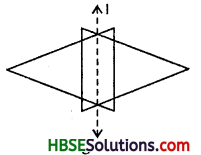# HBSE 6th Class Maths Solutions Chapter 13 Symmetry Ex 13.1

Haryana State Board HBSE 6th Class Maths Solutions Chapter 13 Symmetry Ex 13.1 Textbook Exercise Questions and Answers.

## 13.1

Question 1.
List any four symmetrical object from your home or school.
Solution:
(i) Divider in the mathematical instrument box.
(ii) A bucket in the bathroom.
(iii) An open text book in the middle.
(iv) A closed lock.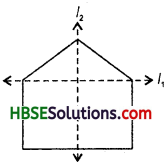Question 2.
For the given figure, which one is the mirror line, l1, or l2 ?
Solution:
Line l2 is the mirror line for the given figure.

Question 3.
Identify the shapes given below.
Check whether they are symmetric or not. Draw the line of symmetry as well.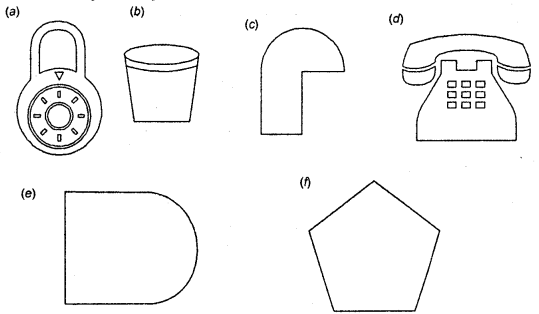Solution:
(a) Lock: Symmetrical,
(b) Bucket: Symmetrical.
(c) Hook : Not symmetrical.
(d) Telephone : Symmetrical.
(e) A field : Symmetrical.
(f) Pentagon: Symmetrical.Question 4.
Copy the following on a squares paper. A square paper is what you would have used in your arithmetic notebook in earlier classes. Then complete them such that the dotted line is the line of symmetry.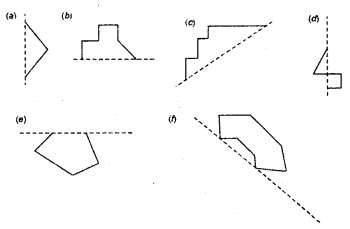Solution: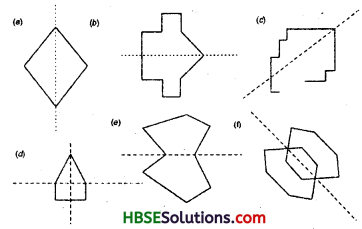Question 5.
In the figure, l is the line of symmetry. Complete the diagram to be symmetric.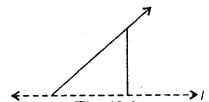Solution: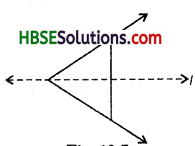Question 6.
In the figure, l is the line of symmetry. Draw the image of the triangle and complete the diagram. So that it becomes symmetric.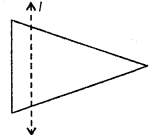Solution: